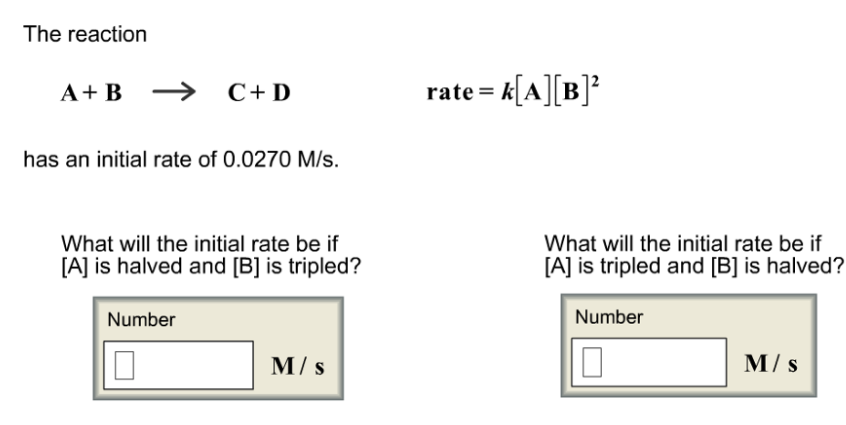# Problem: The reactionA + B → C + Drate = k[A][B]2 has an initial rate of 0.0270 M/s.What will the initial rate be if [A]is halved and [B] is tripled?What will the initial rate be if [A]is tripled and [B] is halved?

###### FREE Expert Solution
93% (42 ratings)###### Problem Details

The reaction

A + B → C + D

rate = k[A][B]

has an initial rate of 0.0270 M/s.

What will the initial rate be if [A]is halved and [B] is tripled?

What will the initial rate be if [A]is tripled and [B] is halved?